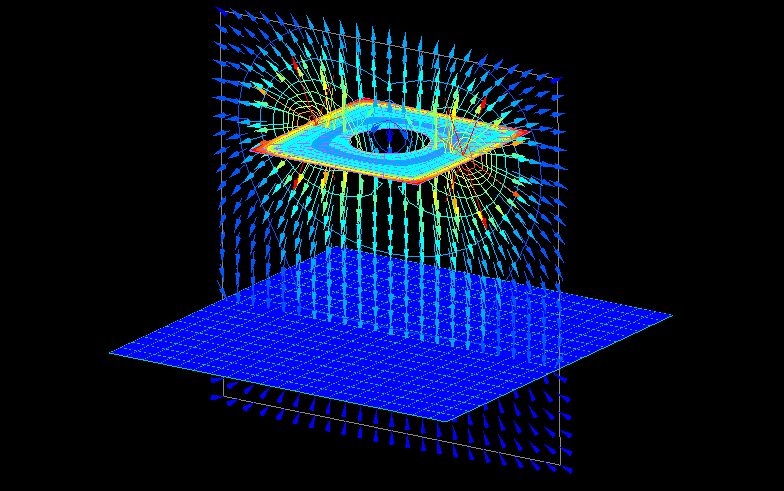Solver Electric Field Analysis ELFIN ≫

The following calculation times are on a Core i7 6700K PC (in seconds).

#### Spatial Electric Field CalculationThis is an example of spatial electric field calculation.
Two square electrodes of different sizes are placed opposite each other.
The smaller electrode has a circular hole and is given 100V.
The larger electrode is set to 0V.

Calculation time: < 1 secondThe spatial electric field is represented by a vector and contour map.
The contour map of an electrode represents the charge density.
Charges are concentrated at the edges of the electrodes so that 100V is achieved at all places of the small electrodes.

#### Two doughnut-shaped electrodes

Calculation time: 6 seconds (Core i7 9700K)Two electrodes are given 1000V and -1000V.
The number of elements of the electrodes is 9600.

#### Three spherical electrodes

Calculation time: 9 seconds (Core i7 9700K)Three electrodes are given 0V, 1000V and 1000V from left to right.
The number of elements of the electrodes is 12000.

#### variable condenser

Calculation time: 11 secondsThis is an example of a variable capacitor analysis.
The calculation is performed by varying the angle of rotation on one side of the disk-shaped parallel electrode.
A dielectric is placed between the parallel electrodes.

#### leaf electroscope

Calculation time: < 1 secondThis is an example of an analysis of a leaf electroscope.
The potential of the charged body (sphere) is varied to calculate the potential of the leaf electroscope.
The forces acting on the leaf are calculated and displayed as contour maps.

#### Current Calculation

Calculation time: < 1 secondThis is an example of analysis of current calculation.
The conductivity of a conductor is replaced by the dielectric constant of a dielectric.
Electric flux density can be converted to current density, and electric charge can be converted to current quantity.

In addition, such curved shape models can be easily created using poly elements.

#### power-transmission line tower

Calculation time: 2 secondsThis is an example of calculating the spatial potential and spatial electric field around a power-transmission line tower.
The voltage applied to each wire is varied slightly to give a pseudo-three-phase alternating current for calculation.
You can see how the electric potential and electric field around each power-transmission line changes over time. (The figure shows the electric field.)

a:12482 t:2 y:0